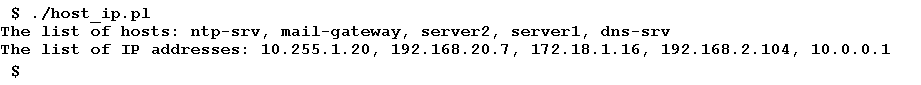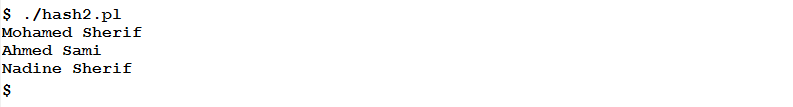# Learn about Hashes in Perl Programming

0
2521Perl supports three main types of variables: Scalars, Arrays, and Hashes. We have already talked about the first two types: Scalars, and Arrays. Now, it is time to talk about the third type: Hashes. An interesting topic to read; so, enjoy!

What are Hashes?
In its simplest definition: a hash is a collection of key-value pairs. Formerly, hashes were named Associative Arrays because they associate each key with its corresponding value. One can think of a hash like an array, in which a value is referenced (indexed) by a unique string called key, instead of being referenced by an integer number (as in arrays).

When to Use Hashes?
Whenever two pieces of data are linked together, so that either one could always lead to the other, they can be considered a key-value pair. Common examples for such a relation are:

• Country, and its Capital.
• Student Name, and his Bench Number.

Initializing Hashes

```%HASHNAME = (“KEY1”, VALUE1, “KEY2”, VALUE2…);

Or:

%HASHNAME = (
“KEY1” => VALUE1,
“KEY2” => VALUE2,
...
“KEYn” => VALUEn
);
```

Where:
HASHNAME is the name of the hash. It could be any valid Perl identifier. Hashes are preceded with % sign.
KEY1 through KEYn are unique strings. An individual key can be used to reference one (and only one) value; that is why I wrote “unique” in bold and underlined.
VALUE1 through VALUEn are scalar values. For a given string key, the corresponding value could be a string, number, or undef.

Example
The following statement initializes a hash named %hosts that contains hostname to IP address mappings:

```%hosts = (      "server1" => "192.168.2.104",
"server2" => "172.18.1.16",
"dns-srv" => "10.0.0.1",
"ntp-srv" => "10.255.1.20",
"mail-gateway" => "192.168.20.7"
);
```

As we agreed in the beginning of our talk, a hash value is referenced for reading or modifying by its key. Using the above hash %hosts, to get the IP address (value) corresponding to the host named “dns-srv” (key), and assign it to the variable \$ip1, the following statement is used:

`\$ip1 = \$hosts{"dns-srv"};`

To modify the value (IP address) corresponding to the hostname “server1” (key):

`\$hosts{"server1”} = “172.30.0.11”;`

Extracting List of Keys
The list of keys in hash can be obtained using the keys() function. This function takes a hash as an argument, and returns an array containing the required list.Extracting List of Values
Similarly, Perl has a function to extract values from a hash. The values() function returns an array containing values in a given hash.

Example
The following script prints the keys and values of the %hosts hash as comma-separated lists.

```#!/bin/perl
%hosts = (      "server1" => "192.168.2.104",
"server2" => "172.18.1.16",
"dns-srv" => "10.0.0.1",
"ntp-srv" => "10.255.1.20",
"mail-gateway" => "192.168.20.7"
);
@h = keys (%hosts);
@IPs = values (%hosts);
print("The list of hosts: ", join(', ', @h), "\n");
print("The list of IP addresses: ", join (', ', @IPs), "\n");
```

When executed, we should get the following output:Obtaining Hash Length
The length (or size) of a hash is the number of elements (key-value pairs) in the hash. It can be easily calculated by obtaining the length of the keys or values array for that hash.

So, to obtain and print the length of the %hosts hash, we could use the following statement:

`print ("Number of Hosts: ", scalar(keys(%hosts)), "\n");`

This should print 5.

`Number of Hosts: 5`

Adding new key-value pairs to a hash is as simple as assigning a value to an existing key. To add the pair “web-srv”, “172.20.10.101” to the %hosts hash:

`\$hosts{"web-srv"} = "172.20.10.101";`

Deleting a Hash Element
To delete an element from a non-empty hash, say hello to the delete() function. Given a hash and key, the function removes the key and the value associated with it from the hash.

Example

```#!/bin/perl
%students = (   "Mohamed Sherif" => 11712,
"Ahmed Sami" => 10135,
"Hussein Taha" => 9846
);
delete (\$students{"Hussein Taha"});
foreach (keys(%students))
{
print (\$_ , "\n");
}
```

When executed, the program should print the keys in the students hash after deleting the pair with key “Hussein Taha”.Summary
In this article, we have learned the third type of variables: Hashes.

• Hashes (formerly known as associative arrays) are collections of key-value pairs.
• A key is a unique string, while the value could be any scalar value.
• Hash names are preceded by %

In the next article, we will start talking about the star of this series: Pattern Matching.